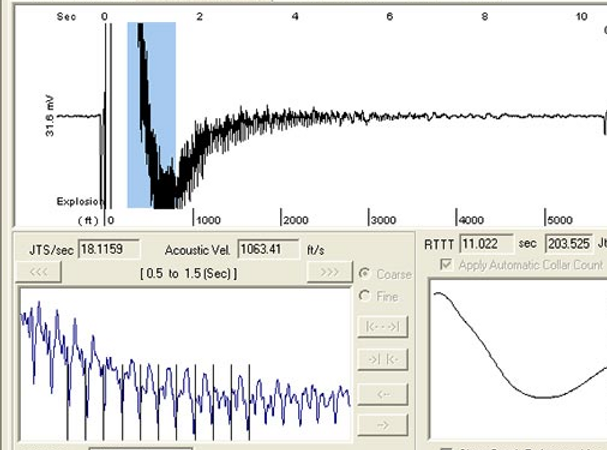Casing Pressure - A quick lookFluid Level analysis should answer the following questions:Match the number of the question with the Red numbers in the example figure below.

• Does Liquid exist above my pump? (you can also subtract 2. from 8. = ft. of liquid above pump intake)
• Depth to top of liquid column?
• What is the maximum production rate available from well?
• What is the percentage of liquid in the Annular Fluid Column?
• Is gas flowing up the Annulus?
• At what rate?
• What is the Gas Gravity?

A quick look at the Depth Determination page.